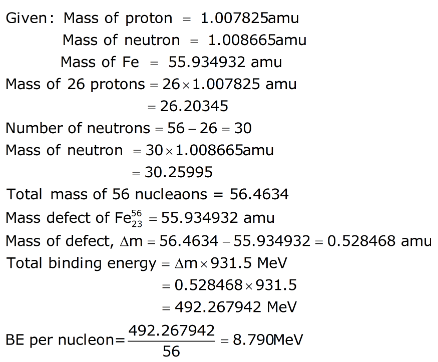# Calculate the binding energy and binding energy per nucleon of 26Fe

calculate the binding energy and binding energy per nucleon of 26Fe raise to 56 nucleus.
given, Mass of proton = 1.007825amu
Mass of neutron = 1.008665amu
Mass of Fe = 55.934932 amu